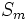# Permutation IAPS

This article describes a particular IAPS of groups, or family of such IAPSes parametrized by some structure

## Definition

### Symbol-free definition

The permutation IAPS is an IAPS of groups where the$n^{th}$ member is the symmetric group$S_n$, and where the block concatenation map$S_m \times S_n \to S_{m+n}$ is defined as the permutation that permutes the first$m$ symbols according to the permutation in$S_m$ and the next$n$ symbols according to the permutation in$S_n$.

### Definition with symbols

The permutation IAPS is an IAPS of groups where the$n^{th}$ member is$S_n$ and the block concatenation map$\Phi_{m,n}: S_m \times S_n \to S_{m+n}$ is defined as follows:

Given a permutation$g \in S_m$ and a permutation$h \in S_n$, the permutation$\Phi_{m,n}(g,h)$ is defined as the following permutation on$\{1,2,3,\dots,m+n\}$. It sends$i \in \{ 1,2,3,\dots,m \}$ to$g(i)$, and sends$j \in \{m + 1, m+2, \dots,m+n\}$ to$h(j-m) + m$.

## Examples

As an example, consider$m = 3$,$n = 4$. Let$g = (1,3)$, and$h = (1,3,4)$. Then:$\Phi_{3,4}(g,h) = (1,3)(4,6,7)$